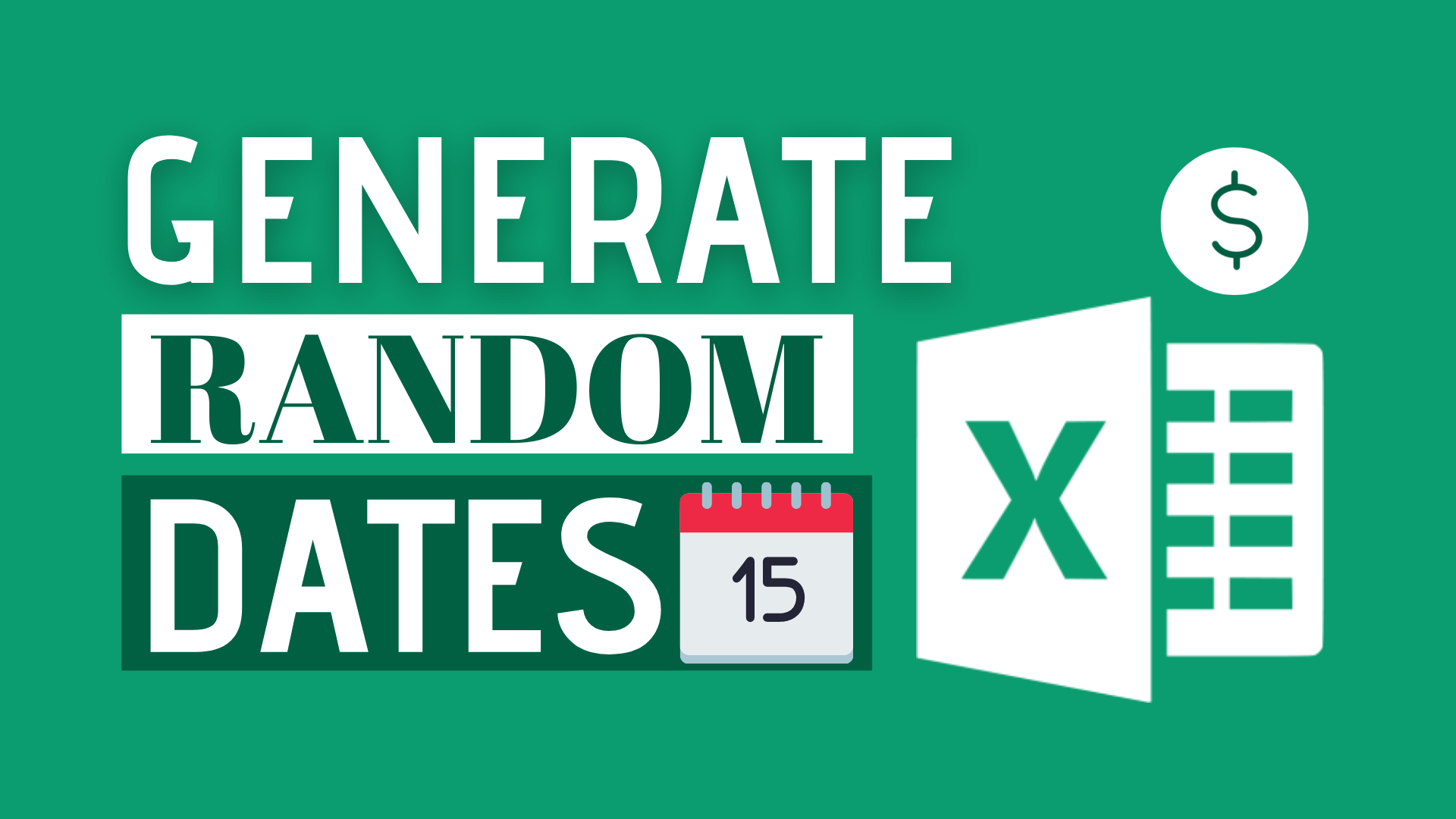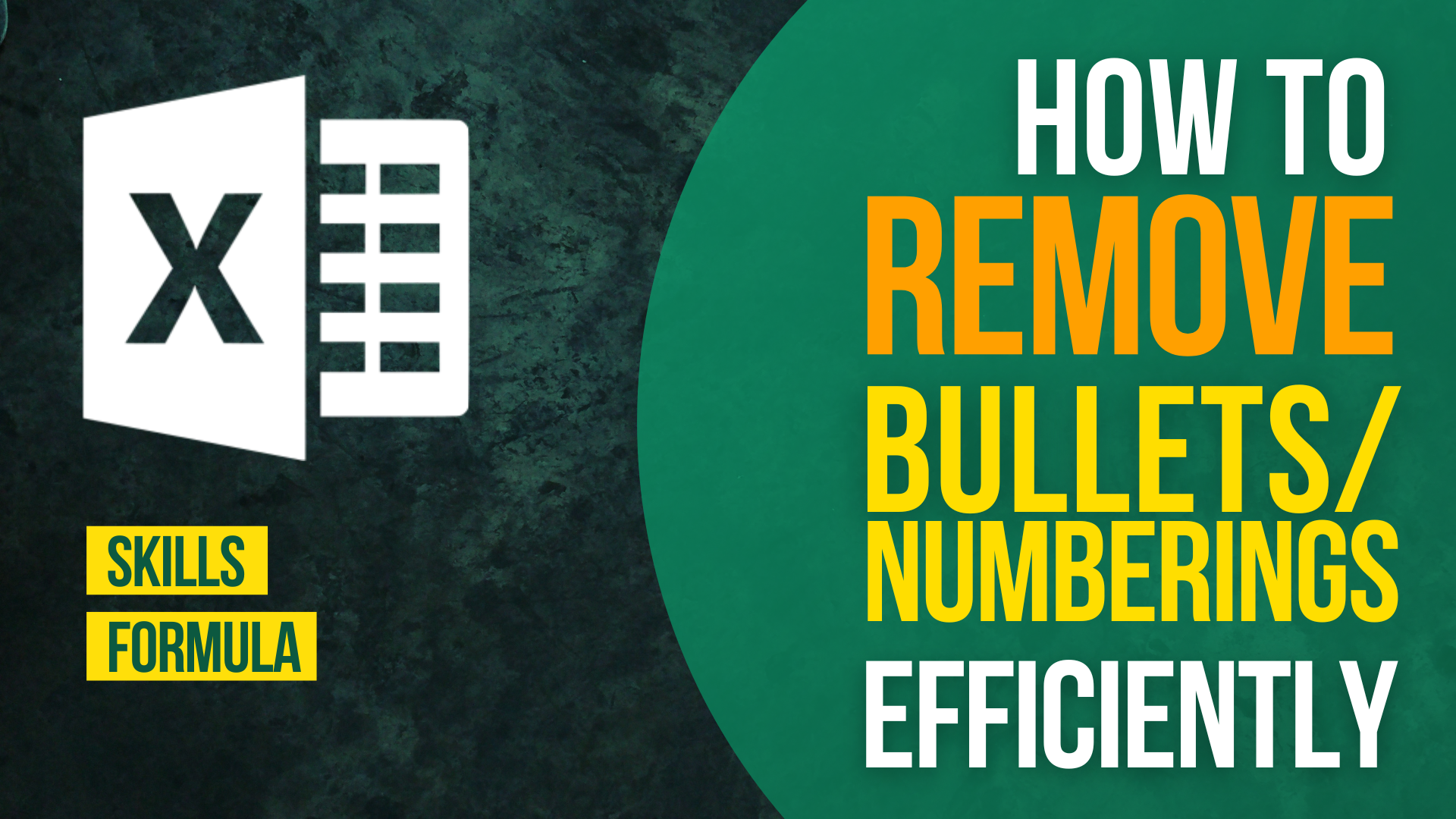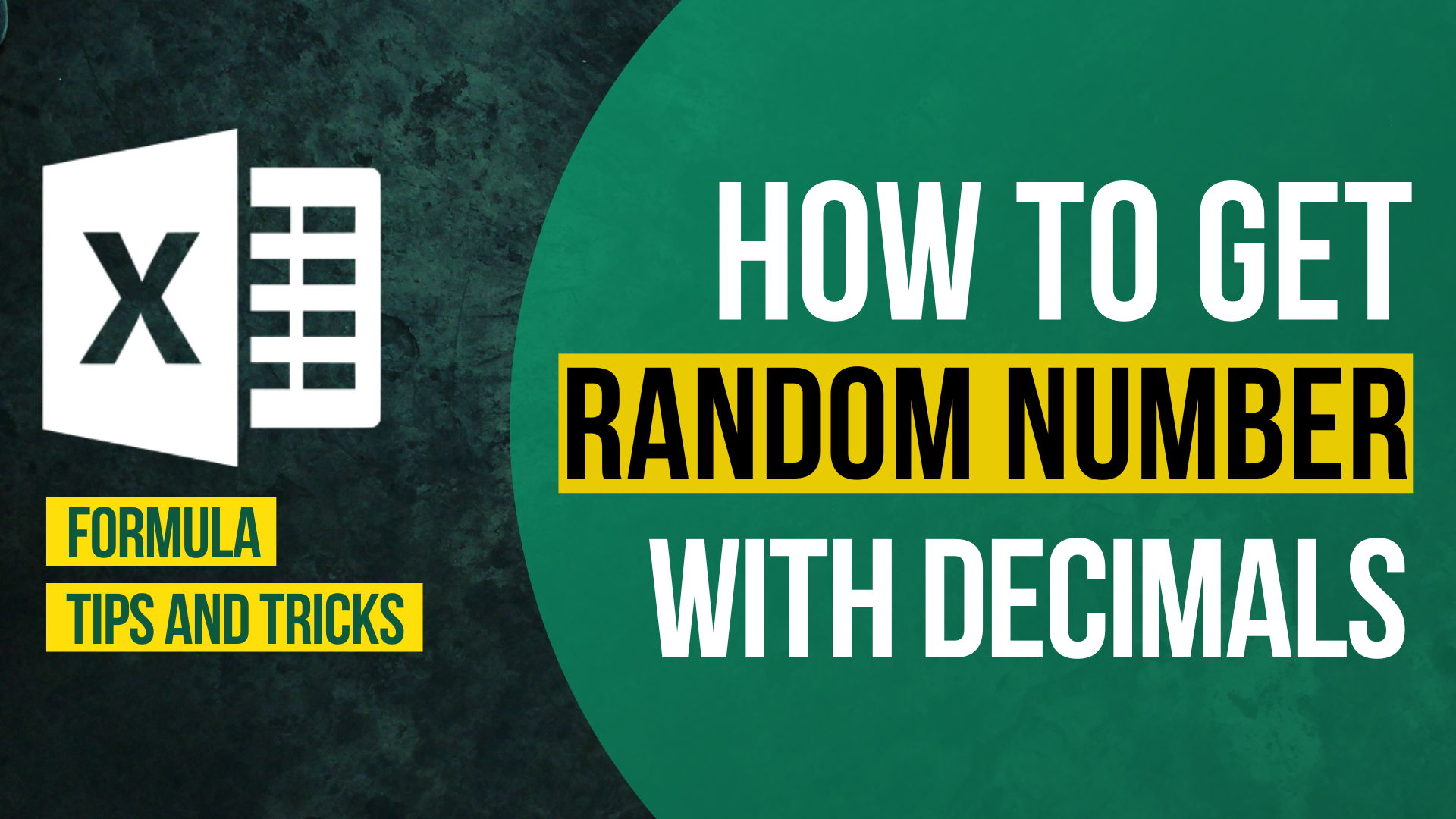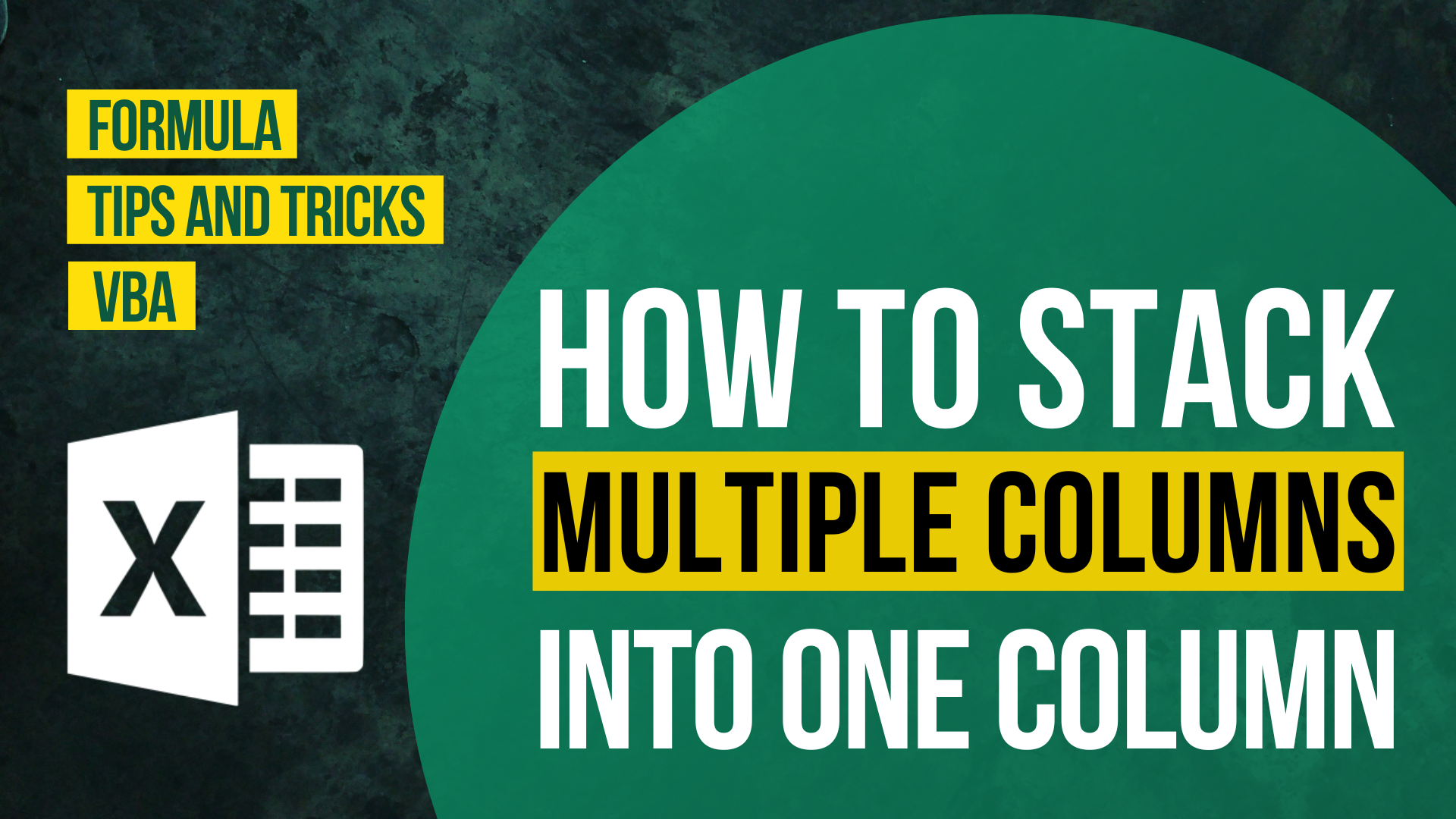# How to Generate Random Dates in Excel## Background

Sometimes we will need to generate random dates in Excel for different reasons.

For example, we may create a set of fake data for practising or predicting.

Before I was aware of this formula, I used to input different dates manually.

Generating random dates in Excel is actually ridiculously simple.

All you need is a formula with two popular functions.

In this article, I will show you how to generate random dates in Excel using RANDBETWEEN() and DATE().

## Steps to Generate Random Dates in Excel

Step 1: Think of a start date and end date for your random dates.

For example, I want my set of dates to fall between 2019/3/4 to 2020/12/4.

Step 2: Customise your formula by changing the start date and end date in the formula

`=RANDBETWEEN(DATE(2019,3,4),DATE(2020,12,4))`

After you entered the formula, your cell will look like this. Instead of a date, Excel gives you a 5-digit number.

No need to panic! That doesn’t mean you encounter an error.

Excel stores date as a number called date serial number. The first date serial number starts at 1900/1/1.

Your number will look just right after formatting it properly.

Step 3: Press Ctrl + Shift + 3 to format your cell as a date

10 Excel Ctrl Shift Shortcuts You Need To Know

Step 4: Done

Many Excel users know that they can generate random numbers using RANDBETWEEN().

But the use of RANDBETWEEN() to generate random dates is so underrated.How to Input Time Duration in ExcelHow to Remove Bullets/Numberings in Excel

Popular posts